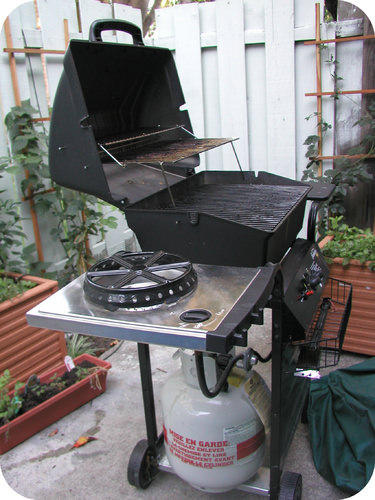# 12.5: Volume-Volume Stoichiometry

$$\newcommand{\vecs}{\overset { \rightharpoonup} {\mathbf{#1}} }$$ $$\newcommand{\vecd}{\overset{-\!-\!\rightharpoonup}{\vphantom{a}\smash {#1}}}$$$$\newcommand{\id}{\mathrm{id}}$$ $$\newcommand{\Span}{\mathrm{span}}$$ $$\newcommand{\kernel}{\mathrm{null}\,}$$ $$\newcommand{\range}{\mathrm{range}\,}$$ $$\newcommand{\RealPart}{\mathrm{Re}}$$ $$\newcommand{\ImaginaryPart}{\mathrm{Im}}$$ $$\newcommand{\Argument}{\mathrm{Arg}}$$ $$\newcommand{\norm}{\| #1 \|}$$ $$\newcommand{\inner}{\langle #1, #2 \rangle}$$ $$\newcommand{\Span}{\mathrm{span}}$$ $$\newcommand{\id}{\mathrm{id}}$$ $$\newcommand{\Span}{\mathrm{span}}$$ $$\newcommand{\kernel}{\mathrm{null}\,}$$ $$\newcommand{\range}{\mathrm{range}\,}$$ $$\newcommand{\RealPart}{\mathrm{Re}}$$ $$\newcommand{\ImaginaryPart}{\mathrm{Im}}$$ $$\newcommand{\Argument}{\mathrm{Arg}}$$ $$\newcommand{\norm}{\| #1 \|}$$ $$\newcommand{\inner}{\langle #1, #2 \rangle}$$ $$\newcommand{\Span}{\mathrm{span}}$$Figure $$\PageIndex{1}$$ (Credit: User:Fluffball70/Wikipedia; Source: http://commons.wikimedia.org/wiki/File:Gasbbq.JPG; License: Public Domain)

### How much propane is left in the tank?

As the weather gets warmer, more and more people want to cook out on the back deck or back yard. Many folks still use charcoal for grilling because of the added flavor. But increasing numbers of back yard cooks like to use a propane grill. The gas burns clean, the grill is ready to go as soon as the flame is lit – but how do you know how much propane is left in the tank? You can buy gauges at hardware stores that measure gas pressure and tell you how much is left in the tank.

## Volume-Volume Stoichiometry

Avogadro's hypothesis states that equal volumes of all gases at the same temperature and pressure contain the same number of gas particles. Further, one mole of any gas at standard temperature and pressure ($$0^\text{o} \text{C}$$ and $$1 \: \text{atm}$$) occupies a volume of $$22.4 \: \text{L}$$. These characteristics make stoichiometry problems involving gases at STP very straightforward. Consider the reaction of nitrogen and oxygen gases to form nitrogen dioxide:

$\begin{array}{lllll} \ce{N_2} \left( g \right) & + & 2 \ce{O_2} \left( g \right) & \rightarrow & 2 \ce{NO_2} \left( g \right) \\ 1 \: \text{molecule} & & 2 \: \text{molecules} & & 2 \: \text{molecules} \\ 1 \: \text{mol} & & 2 \: \text{mol} & & 2 \: \text{mol} \\ 1 \: \text{volume} & & 2 \: \text{volumes} & & 2 \: \text{volumes} \end{array}\nonumber$

Because of Avogadro's work, we know that the mole ratios between substances in a gas-phase reaction are also volume ratios. The six possible volume ratios for the above equation are:

\begin{align*} \frac{1 \: \text{volume} \: \ce{N_2}}{2 \: \text{volumes} \: \ce{O_2}} &\text{or} \frac{2 \: \text{volumes} \: \ce{O_2}}{1 \: \text{volume} \: \ce{N_2}} \\ \frac{1 \: \text{volume} \: \ce{N_2}}{2 \: \text{volumes} \: \ce{NO_2}} &\text{or} \frac{2 \: \text{volumes} \: \ce{NO_2}}{1 \: \text{volume} \: \ce{N_2}} \\ \frac{2 \: \text{volumes} \: \ce{O_2}}{2 \: \text{volumes} \: \ce{NO_2}} &\text{or} \frac{2 \: \text{volumes} \: \ce{NO_2}}{2 \: \text{volumes} \: \ce{O_2}} \end{align*}\nonumber

The volume ratios above can easily be used when the volume of one gas in a reaction is known, and you need to determine the volume of another gas that will either react with or be produced from the first gas. The pressure and temperature conditions of both gases need to be the same.

Example $$\PageIndex{1}$$: Volume-Volume Stoichiometry

The combustion of propane gas produces carbon dioxide and water vapor.

$\ce{C_3H_8} \left( g \right) + 5 \ce{O_2} \left( g \right) \rightarrow 3 \ce{CO_2} \left( g \right) + 4 \ce{H_2O} \left( g \right)\nonumber$

What volume of oxygen is required to completely combust $$0.650 \: \text{L}$$ of propane? What volume of carbon dioxide is produced in the reaction?

Solution:

Step 1: List the known quantities and plan the problem.

Known

• Given: $$0.650 \: \text{L} \: \ce{C_3H_8}$$
• 1 volume $$\ce{C_3H_8} = 5$$ volumes $$\ce{O_2}$$
• 1 volume $$\ce{C_3H_8} = 3$$ volumes $$\ce{CO_2}$$

Unknown

• Volume $$\ce{O_2} = ? \: \text{L}$$
• Volume $$\ce{CO_2} = ? \: \text{L}$$

Two separate calculations can be done using the volume ratios.

Step 2: Solve.

$0.650 \: \text{L} \: \ce{C_3H_8} \times \frac{5 \: \text{L} \: \ce{O_2}}{1 \: \text{L} \: \ce{C_3H_8}} = 3.25 \: \text{L} \: \ce{O_2}\nonumber$

$0.650 \: \text{L} \: \ce{C_3H_8} \times \frac{3 \: \text{L} \: \ce{CO_2}}{1 \: \text{L} \: \ce{C_3H_8}} = 1.95 \: \text{L} \: \ce{CO_2}\nonumber$

Because the coefficients of the $$\ce{O_2}$$ and the $$\ce{CO_2}$$ are larger than that of the $$\ce{C_3H_8}$$, the volumes for those two gases are greater. Note that total volume is not necessarily conserved in a reaction because moles are not necessarily conserved. In this reaction, 6 total volumes of reactants become 7 total volumes of products.

## Summary

• Calculations of volume-volume ratios are based on Avogadro's hypothesis.
• Pressures and temperatures of the gases involved need to be the same.